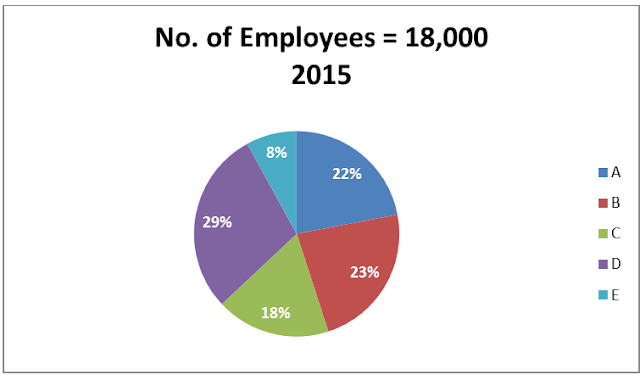# Quantitative Aptitude Practice Questions for SBI PO

Quantitative Aptitude Practice Questions for SBI PO
Directions (1 – 5): The following pie charts give the percentage distribution of employees in different departments in any company during the years 2015 and 2016 .Answer the questions using this data.
A-Administration;  B-Operations; C-Sales& Marketing; D-Finance & Accounts;  E-Corporate HQ1. If the average monthly salary of employees in Administration was rs.15000 in 2015,what was the total salary expense of Administration on 2015 (in crore)?
a) 71.28
b) 57.45
c) 78.98
d) 64.89
e) 59.4
2. What is the percentage increase/decrease in the number of employees in Finance &  Accounts ?
a) 23.37 % increase
b) 23.37% decrease
c) 34.35 % increase
d) 34.35 % decrease
e) no change
3. Find the ratio of no of employees in department E in 2015 to the no.of employees in department D in 2016.
a) 3:5
b) 9 : 25
c) 4:5
d) can't be determined
e) none of these
4. If 300 employees left Operations at the end of 2015, how many joined in 2016?
a) 480
b) 960
c) 1360
d) 1540
e) 1240
5. Find the average no. of employees of Corporate HQ in 2015 and 2016.
a) 1520
b) 1730
c) 1650
d) 1450
e) 1720
Directions (6 – 10): Study the following graph and answer the question based on it-
Monthly Expenditure (in thousands) by five people on Rent, Food, Children's Education, Cloths and Travelling
6. What is the total monthly expenditure made by Megha on rent, Tarun on clothes and Aarushi on travelling together?
a) Rs. 15520
b) Rs. 16120
c) Rs. 17820
d) Rs. 18320
e) Rs. 19420
7. What is the average monthly expenditure on food by all the people together?
a) Rs. 8250
b) Rs. 9550
c) Rs. 9950
d) Rs. 10160
e) Rs. 10850
8. Whose monthly expenditure on all the heads together is the lowest among them?
a) Seema
b) Megha
c) Tarun
d) Varun
e) Aarushi
9. If the monthly expenditure of Seema on children's education is increased by 5%, then what will be his yearly expenditure on children's education?
a) Rs. 102760
b) Rs. 134760
c) Rs. 141760
d) Rs. 158760
e) Rs. 160760
10. What is the respective ratio between the monthly expenditure made by Varun on travelling and the monthly expenditure made by Megha on clothes?
a) 39 : 95
b) 95 : 39
c) 59 : 105
d) 105 : 59
e) Can’t be determine
Solutions:
1. A) total monthly salary=18000×0.22×15000=5.94 crore
total annual salary=5.94×12=71.28 crore
2. B) in 2015 = 5220; in 2016 = 4000
% decrease = 1220/5220 × 100 = 23.37%
3. B) Required ratio = 18000 × 8/100 : 20000 × 20/100 = 9 : 25
4. C) The no. of joiners would be given by =5200-(4140-300)=1360
5. E) Required Average = (8% of 18000 + 10% of 20000) = (1440 + 2000)/2 = 3440/2 = 1720
6. C) Required monthly expenses = Rs. (9.65 + 2.75 + 5.42) thousand = Rs. (17.82 x 1000) = Rs. 17820
7. E) Monthly expenditure on food = Rs. (7.50 + 8.55 + 11.40 + 17.80 + 9)/5 thousand = Rs. 10.85 thousand = Rs. 10850
8. D)
9. D) Required annual expenditure of Seema on education
= Rs. (12 × 12.60 × 105)/100 thousand = Rs. 158.76 thousand = Rs. 158760
10. C) Required ratio = 4.72 : 8.40 = 472 : 840 = 59 : 105